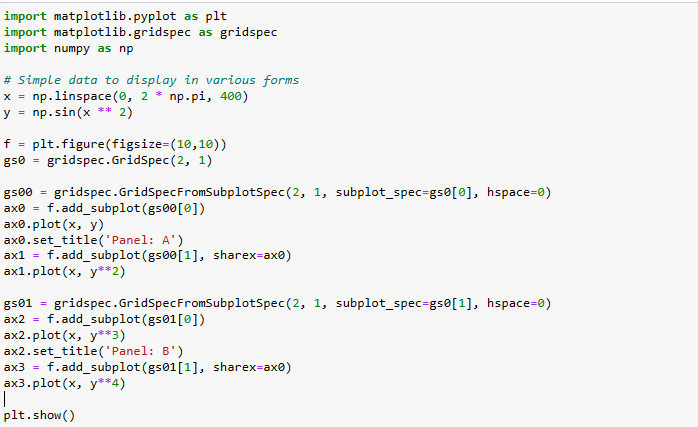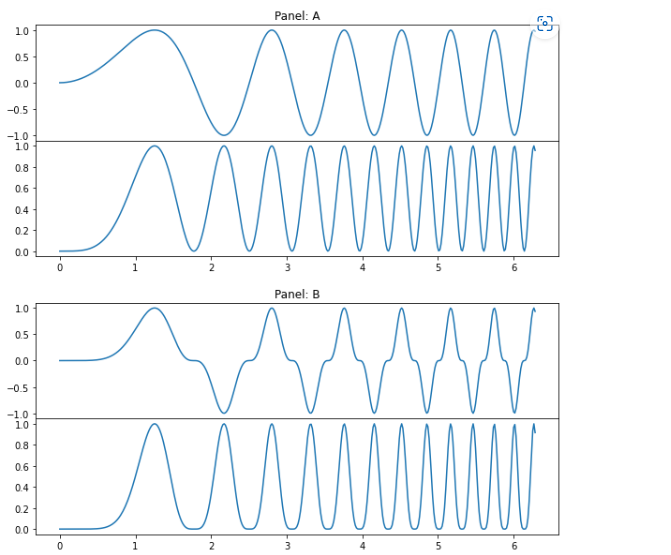# Set the spacing between subplots in Matplotlib Pythonby kanikaUpdated: May 8, 2023Solution Kit

Matplotlib subplots are a great way to create many plots in the same figure. Subplots allow you to arrange many plots with different x- and y-axes into a single figure.

### Creating Subplots:

To create a new subplot in matplotlib, you can use the following syntax:

fig, ax = plt.subplots(nrows=1, ncols=1)

Here, nrows and ncols indicate the subplot grid's number of rows and columns. This will create a single subplot in the figure. You can specify the nrows and ncols arguments to create many subplots.

### Customizing Subplots:

Once you have created a subplot in matplotlib, you can customize its appearance. We can do it using the various functions and methods in the matplotlib library. You can customize the x- and y-axes of the subplot, change its size, add titles and labels, and more. For example, you can use the set_xlabel() and set_ylabel() methods to add labels to the x- and y-axes of the subplot. You can also use the set_title() method to add a title to the subplot. Using the figsize argument, you can customize the subplot size. This argument takes a tuple of two values, indicating the width and height of the subplot in inches.

We can create several types of plots with matplotlib are:

1. Bar Plots:

Bar plots represent categorical data created by plotting bars representing different values' frequencies.

2. Histograms:

Histograms represent continuous data. We can create it by plotting bars representing different intervals' frequencies.

3. Scatter Plots:

It displays the relationship between two variables. We can create it by plotting points representing each variable's values.

4. Line Plots:

Line plots help show how one variable changes over time. We can do it by plotting a line that connects the values of the variable.

5. Pie Charts:

Pie charts represent the proportions of distinct categories. We can do it by plotting wedges representing each category's relative proportions.

6. Box Plots:

Box plots represent the spread of data. We can do it by plotting a box representing the data's quartiles.

7. Contour Plots:

Contour plots represent three-dimensional data. We can create it by plotting curved lines. It will represent the values of the data at different points in a three-dimensional space.

We can plot the data on a subplot using various methods. We can plot a simple text by specifying the text string, font size, and color. We can generate bar charts, line graphs, and scatter plots with a few lines of code. More complex representations, like contour and 3D plots. We can create with the help of numerical libraries such as NumPy and Matplotlib. Additionally, we can use mathematical formulas. It helps generate histograms, probability distributions, and density plots.

It can improve your analysis skills, from creating visualizations to complex statistical analyses. Start with a basic subplot to generate visualizations of your data. For example, you can use a simple line plot to see trends or correlations between variables. Consider adding extra subplots to compare different aspects of your data. For example, you could create box plots to visualize the distribution of your data better. Use a combination of subplots to compare variables across different periods. For example, you could use a line plot, a bar plot, and a scatter plot to compare the trends of different variables. Use the layout capabilities of matplotlib to arrange your subplots.

For example, you could arrange your subplots in a grid. You can create an organized visualization. Use Matplotlib's statistical analysis capabilities to gain deeper insights into your data. For example, you could use the correlation function. It helps explore the relationship between variables. Consider adding extra features to your subplots. The features can be legends, labels, annotations, and colors. It helps make them easier to interpret.

Some of the unique aspects of the matplotlib plotting library are:

• Many Plots on a Single Figure:

Matplotlib allows users to plot many plots on the same figure. It helps make it easy to compare data or to create a more complex figure with many plot types.

• Different Plot Types:

Matplotlib can create various plot types. The types include line plots, bar charts, histograms, and scatter plots.

• Customizable Legends:

We can customize the legends, allowing for easy labeling and comparison of data.

• Subplots:

Subplots are a wonderful way to view many plots. Matplotlib provides a straightforward way to create them.

• Subplot Layout Customization:

Matplotlib also allows users to customize the layout of their subplots. We can do it by allowing for more flexibility when creating complex figures.

• Annotations:

We can add the annotations to figures in Matplotlib. We can provide an effortless way to highlight important points in a plot.

• 3D Plots:

Matplotlib can also create 3D plots, allowing users to visualize data in a new way.Fig1: Preview of the Code.Fig1: Preview of the Output.

### Code

In this solution, we are setting the space between the subplots.

### Instructions

Follow the steps carefully to get the output easily.

1. Install Jupyter Notebook on your computer.
2. Open terminal and install the required libraries with following commands.
3. Install numpy - pip install numpy.
4. Install matplotlib - pip install matplotlib.
5. Copy the code using the "Copy" button above and paste it into your IDE's Python file.
6. Run the file.

I hope you found this useful. I have added the link to dependent libraries, version information in the following sections.

I found this code snippet by searching for "How to set the spacing between subplots in Matplotlib Python" in kandi. You can try any such use case!

### Dependent Libraries

matplotlibby matplotlib

Python17559Version:v3.7.1License: No License (null)

matplotlib: plotting with Python

Support
Quality
Security
Reuse

matplotlibby matplotlib

Python17559Version:v3.7.1License: No License

matplotlib: plotting with Python
Support
Quality
Security
Reuse

numpyby numpy

Python23755Version:v1.25.0rc1The fundamental package for scientific computing with Python.

Support
Quality
Security
Reuse

numpyby numpy

Python23755Version:v1.25.0rc1License: Permissive (BSD-3-Clause)

The fundamental package for scientific computing with Python.
Support
Quality
Security
Reuse

If you do not have matplotlib or numpy that is required to run this code, you can install it by clicking on the above link and copying the pip Install command from the respective page in kandi.

You can search for any dependent library on kandi like matplotlib

### FAQ

What are matplotlib subplots, and how does it work?

Matplotlib Subplots is a function that allows you to plot many figures in one figure. This is useful when we break up a complex graph into smaller components. It works by creating a grid of subplots and then assigning each subplot to a specific figure. You can customize each subplot and combine them into one figure.

How can we use SciPy to adjust the spacing of subplots in matplotlib?

SciPy can adjust the spacing of subplots by using the matplotlib.pyplot.subplots_adjust(). This function takes parameters like left, right, top, bottom, and wspace. It can adjust the spacing between the subplots.

How do I access the subplot tool window within matplotlib?

We can access the subplot tool window using the plt.subplots() command. This command will generate a figure and axes object. It can create subplots within the figure.

Is it necessary to import numpy when using matplotlib subplots?

No, it is not necessary to import NumPy when using Matplotlib subplots. But we use NumPy to perform mathematical operations on data before plotting it.

How can we change the axis labels within a single plot or across many plots in a figure?

Within a single plot:

We can change the axis labels in the plot code or through the plot's object. For example, the xlabel and ylabel functions can rename the x-axis and y-axis labels.

Across many plots in a figure:

We can change the axis labels across many plots in a figure by looping over the subplots. We can rename the xlabel and ylabel functions and the x-axis and y-axis labels for each subplot.

What are some tips for adjusting vertical spacing between subplots in matplotlib?

• Use the plt.subplots_adjust() function to adjust the vertical spacing between subplots. We can use the parameters wspace and hspace to adjust the height and width of the space between subplots.
• Use the fig.tight_layout() function to adjust the spacing between subplots.
• Use the fig.subplots_adjust() function to adjust the margins between the subplots.
• Use the plt.figtext() function to add text to the plot area. We can do it by adding vertical spacing between subplots.
• Use the plt.gcf().subplots_adjust() function to adjust the spacing between subplots. We can do it when using the subplots() function.

Can we combine the individual elements into a single plot with matplotlib subplots?

Yes, we can combine the individual elements from many plots into a single plot with subplots. This function allows you to create a grid of plots where we can manipulate each plot. It can combine them into a single plot.

Are there advantages of using scatter instead of line graphs for visualizing data?

There are advantages to using scatter instead of line graphs for visualizing data. We plot the scatter plots for a more nuanced and detailed data analysis. It is because they allow many variables. Scatter plots also allow for the representation of outliers, which line graphs do not. Additionally, scatter plots are better suited to represent non-linear relationships between data points. It is because line graphs are better suited to represent linear relationships. Finally, scatter plots are better for visualizing large datasets. It is because they allow for more clarity than line graphs.

Can you change the size of your figure when working with many plots at once with MatPlotLib SubPlots?

Yes, it is possible to change the figure size when working with plots with SubPlots. We can change the figure size using the figsize argument when creating the figure. For example, fig = plt.figure(figsize=(8, 6)) would set the figure size to 8x6 inches.

How can you arrange grid columns within a figure when creating many plots?

You can arrange the grid columns using the "ncols" parameter when creating plots. The number you enter for this parameter will determine the number of columns in the grid. For example, if you enter 2, we will arrange the grid with two columns.

### Environment Tested

I tested this solution in the following versions. Be mindful of changes when working with other versions.

1. The solution is created in Python 3.9.6
2. The solution is tested on matplotlib version 3.5.0
3. The solution is tested on numpy version 1.21.4

Using this solution, we are able to create spacing between the subplots.

### Support

1. For any support on kandi solution kits, please use the chat
2. For further learning resources, visit the Open Weaver Community learning page.

See similar Kits and Libraries